# SAT Math Multiple Choice Question 888: Answer and Explanation

### Test Information

Question: 888

3.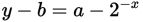For the equation given, if a > 0 and b > 0, then which of the following statements is always true?

• A.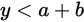• B.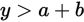• C.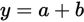• D.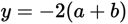Explanation:

A

Difficulty: Hard

Category: Passport to Advanced Math / Exponents

Strategic Advice: Sometimes it is necessary to rearrange an expression or equation to reveal properties about the quantity being defined.

Getting to the Answer: Each of the answer choices compares y to some expression, so rewrite the equation in terms of y by adding b to both sides. Then, group a and b so the equation looks more like the answer choices: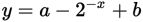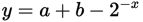Now it's time to examine the information given in the question and some properties of exponents. It is given that a and b are both greater than 0, or in other words, positive numbers. This means a + b must be a positive number as well. The term 2-x is also a positive number because raising a number to a negative power simply means writing the reciprocal of the number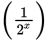. When x is large, this number becomes very small, but it is still a positive number. Therefore, y is equal to a positive number (a + b) minus another positive number. It follows that a + b - 2 -x < a + b, and therefore that y < a + b, which matches (A).